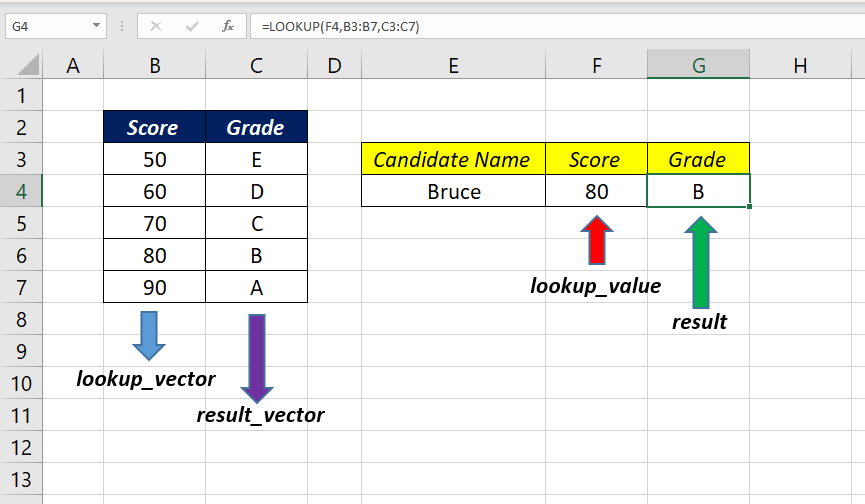# LOOKUP FunctionThe Excel LOOKUP function is a LOOKUP & REFERENCE function that is used to search a value in a single row/column and return the corresponding value from the second row/column.

### Function Type

Lookup and reference

### Vector Form of Lookup Function

LOOKUP function has two forms, Vector and Array. We will see the Vector form first.

Use the vector form of LOOKUP function when you want to specify the range that contains the values that you want to match.

### Purpose

Look for a value in a row/column and return the corresponding value from the second row/column.

### Return value

A value in the result vector.

### Syntax

` =LOOKUP(lookup_value, lookup_vector, [result_vector]) `

### Arguments

lookup_value – The value LOOKUP searches for in the first vector. This can be a number, text, a logical value, or a name or reference that refers to a value

lookup_vector – that one-row, or one-column range to search

result_vector – that one-row, or one-column range containing results. this should be of the same size that of lookup_vector

### Examples

#### Grade from the Score of a Candidate

In this example, we will see how to use the LOOKUP function to return the Grade after analyzing the score of a candidate.

The following formula will return the Grade of the score 80 in the cell F4 after comparing it with the Grade and Score Matrix in the range B3:C7.

`=LOOKUP(F4,B3:B7,C3:C7)`

If the LOOKUP function can’t find an exact match, the function will return the value corresponding to the next smallest value.

In this case, if we search for the Grade of the score 65, LOOKUP function will return the value D

`=LOOKUP(F5,B3:B7,C3:C7)`

LOOKUP function can also perform lookups in the horizontal direction.

In the following example, I have aligned the same data (Grade/Score Matrix) in columns (C3:G4) and used LOOKUP function to find the Grade corresponding to the candidate score in the cells C7 and C8

`=LOOKUP(C8,C3:G3,C4:G4)`

### Array Form of Lookup Function

The array form of LOOKUP function looks in the first row or column of an array for the specified value and returns a value from the same position in the last row or column of the array. This form of LOOKUP function is used when the value that needs to be matched is in the first row or column of the array.

### Purpose

Look for a value in a row/column and return the corresponding value from the second row/column.

### Return value

A value from the last column or row of the array.

### Syntax

`=LOOKUP(lookup_value, array) `

### Arguments

lookup_value – The value LOOKUP searches for in the first vector. This can be a number, text, a logical value, or a name or reference that refers to a value

array – A range of cells that contains text, numbers, or logical values that you want to compare with lookup_value

### Example

#### Perform a lookup for the Country name using Country code

In this example, LOOKUP function is used to return the country Name from a table containing Country Code (B3:B7), ISO Code (C3:C7) and Country Name (D3:D7)

The following formula will look for the Country Code 81 (lookup value in the cell G7) in the range of cells from B3:D7 (array) and will return the corresponding country name, Japan.

`=LOOKUP(G5,B3:D7)`